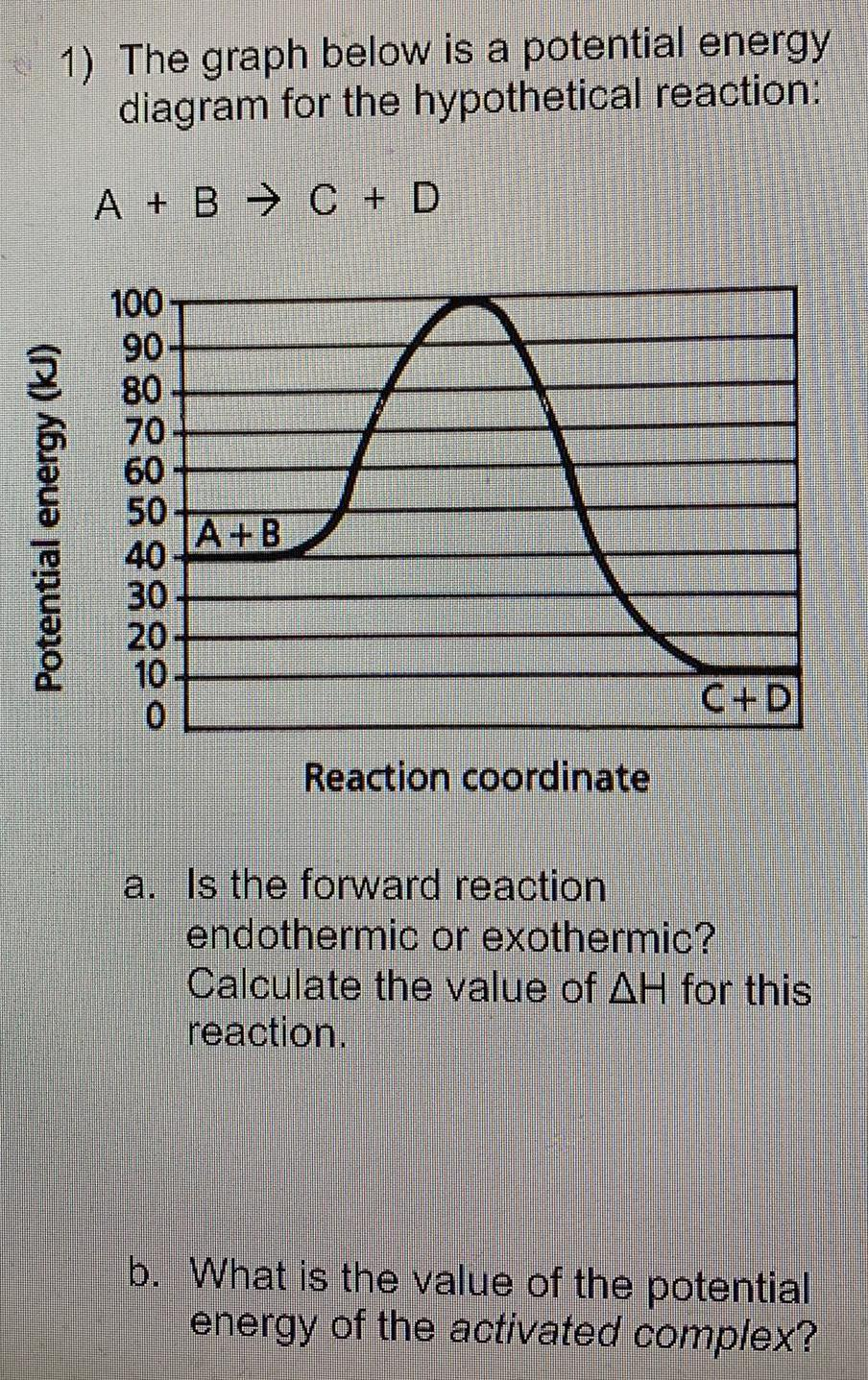Question:

# The graph below is a potential energy diagram for the

Last updated: 7/31/2022The graph below is a potential energy diagram for the hypothetical reaction: A + B - C + D a. Is the forward reaction endothermic or exothermic? Calculate the value of AH for this reaction. b. What is the value of the potential energy of the activated complex?